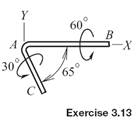### Create an Account

Home / Questions / Consider the rotation of the bent rod in Exercise 313 Determine the orientation of the axi...

# Consider the rotation of the bent rod in Exercise 313 Determine the orientation of the axis and the angle of rotation of the single rotation that would be equivalent to the pair of rotations

Consider the rotation of the bent rod in Exercise 3.13. Determine the orientation of the axis and the angle of rotation of the single rotation that would be equivalent to the pair of rotations specified there.

Exercise 3.13

The bent rod is given a pair of rotations, first by 60° about line AB, and then 30◦ about line AC, with the sense of each rotation as shown in the sketch. Let xyz be a coordinate system fixed to the rod that initially aligned with the fixed XYZ system shown. Determine the transformation by which vector components with respect to XYZ may be converted to components with respect to xyz.Jun 16 2020 View more View LessSubscribe To Get Solution